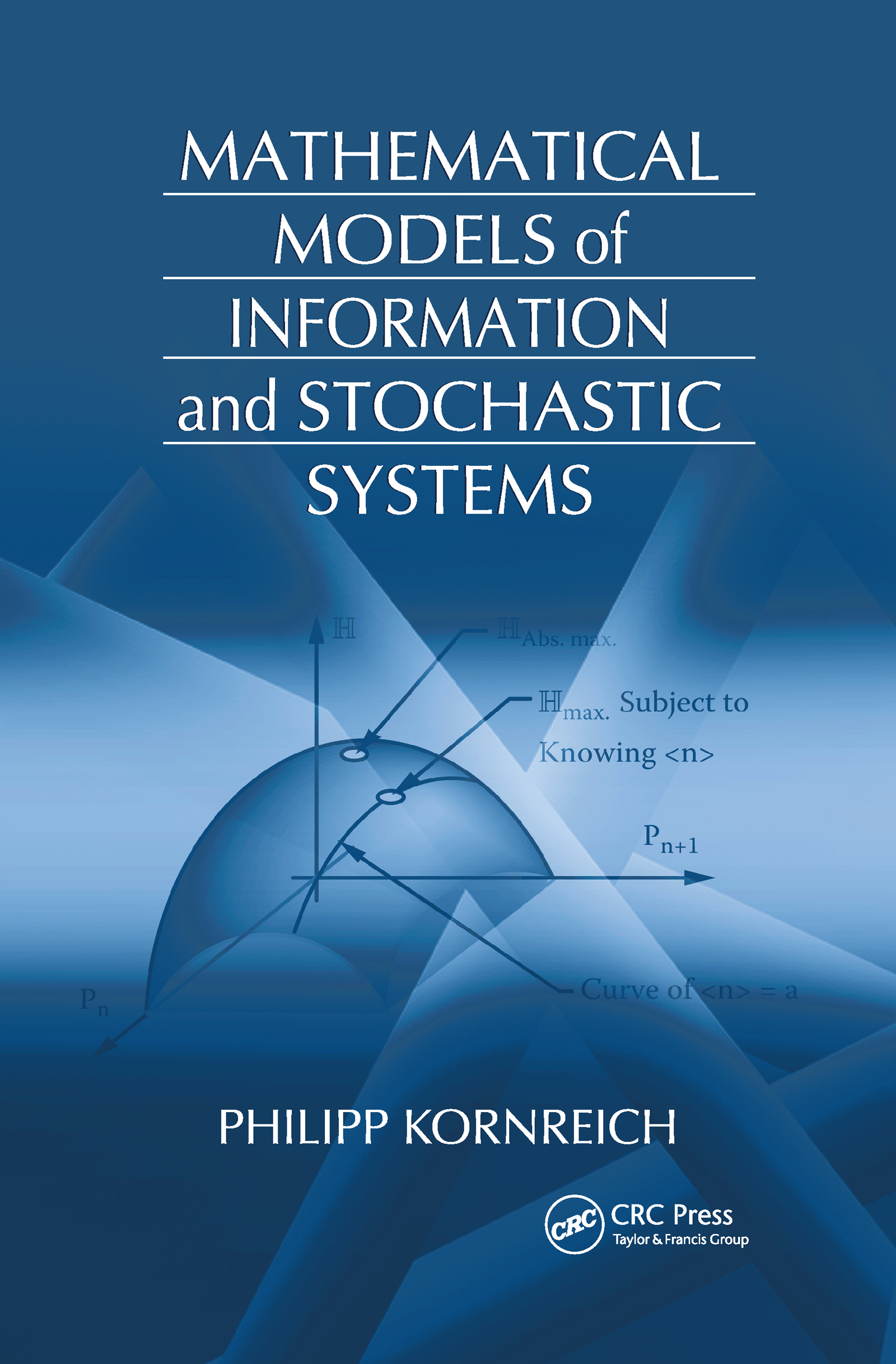Mathematical Models of Information and Stochastic Systems

1st Edition

CRC Press

376 pages | 124 B/W Illus.

Hardback: 9781420058833
pub: 2008-05-13
Currently out of stock
\$140.00
x
eBook (VitalSource) : 9781315219158
pub: 2018-10-03
from \$70.00

FREE Standard Shipping!

Description

From ancient soothsayers and astrologists to today’s pollsters and economists, probability theory has long been used to predict the future on the basis of past and present knowledge. Mathematical Models of Information and Stochastic Systems shows that the amount of knowledge about a system plays an important role in the mathematical models used to foretell the future of the system. It explains how this known quantity of information is used to derive a system’s probabilistic properties.

After an introduction, the book presents several basic principles that are employed in the remainder of the text to develop useful examples of probability theory. It examines both discrete and continuous distribution functions and random variables, followed by a chapter on the average values, correlations, and covariances of functions of variables as well as the probabilistic mathematical model of quantum mechanics. The author then explores the concepts of randomness and entropy and derives various discrete probabilities and continuous probability density functions from what is known about a particular stochastic system. The final chapters discuss information of discrete and continuous systems, time-dependent stochastic processes, data analysis, and chaotic systems and fractals.

By building a range of probability distributions based on prior knowledge of the problem, this classroom-tested text illustrates how to predict the behavior of diverse systems. A solutions manual is available for qualifying instructors.

PREFACE

Introduction

Historical Development and Aspects of Probability Theory

Discussion of the Material in This Text

References

Events and Density of Events

General Probability Concepts

Probabilities of Continuous Sets of Events

Discrete Events Having the Same Probability

Digression of Factorials and the Γ Function

Continuous Sets of Events Having the Same Probability, Density of States

Problems

Joint, Conditional, and Total Probabilities Conditional Probabilities

Dependent, Independent, and Exclusive Events

Total Probability and Bayes’ Theorem of Discrete Events

Markov Processes

Joint, Conditional, and Total Probabilities and Bayes’ Theorem of Continuous Events

Problems

Random Variables and Functions of Random Variables

Concept of a Random Variable and Functions of a Random Variable

Discrete Distribution Functions

Discrete Distribution Functions for More Than One Value of a Random Variable with the Same Probability

Continuous Distribution and Density Functions

Continuous Distribution Functions for More Than One Value of a Random Variable with the Same Probability

Discrete Distribution Functions of Multiple Random Variables

Continuous Distribution Functions of Multiple Random Variables

Phase Space, a Special Case of Multiple Random Variables

Problems

Conditional Distribution Functions and a Special Case: The Sum of Two Random Variables

Discrete Conditional Distribution Functions

Continuous Conditional Distribution Functions

A Special Case: The Sum of Two Statistically Independent Discrete Random Variables

A Special Case: The Sum of Two Statistically Independent Continuous Random Variables

Problems

Average Values, Moments, and Correlations of Random Variables and of Functions of Random Variables

The Most Likely Value of a Random Variable

The Average Value of a Discrete Random Variable and of a Function of a Discrete Random Variable

An Often-Used Special Case

The Probabilistic Mathematical Model of Discrete Quantum Mechanics

The Average Value of a Continuous Random Variable and of a Function of a Continuous Random Variable

The Probabilistic Model of Continuous Quantum Mechanics

Moments of Random Variables

Conditional Average Value of a Random Variable and of a Function of a Random Variable

Central Moments

Variance and Standard Deviation

Correlations of Two Random Variables and of Functions of Random Variables

A Special Case: The Average Value of e−jkx

References

Problems

Randomness and Average Randomness

The Concept of Randomness of Discrete Events

The Concept of Randomness of Continuous Events

The Average Randomness of Discrete Events

The Average Randomness of Continuous Random Variables

The Average Randomness of Random Variables with Values That Have the Same Probability

The Entropy of Real Physical Systems and a Very Large Number

The Cepstrum

Stochastic Temperature and the Legendre Transform

Other Stochastic Potentials and the Noise Figure

References

Problems

Most Random Systems

Methods for Determining Probabilities

Determining Probabilities Based on What Is Known about a System

The Poisson Probability and One of Its Applications

Continuous Most Random Systems

Properties of Gaussian Stochastic Systems

Important Examples of Stochastic Physical Systems

The Limit of Zero and Very Large Temperatures

References

Problems

Information

Information

Information in Genes

Information Transmission of Discrete Systems

Information Transmission of Continuous or Analog Systems

The Maximum Information and Optimum Transmission Rates of Discrete Systems

The Maximum Information and Optimum Transmission Rates of Continuous or Analog Systems

The Bit Error Rate

References

Problems

Random Processes

Random Processes

Random Walk and the Famous Case of Scent Molecules Emerging from a Perfume Bottle

The Simple Stochastic Oscillator and Clocks

Correlation Functions of Random Processes

Stationarity of Random Processes

The Time Average and Ergodicity of Random Processes

Partially Coherent Light Rays as Random Processes

Stochastic Aspects of Transitions between States

Cantor Sets as Random Processes

References

Problems

Spectral Densities

Stochastic Power

The Power Spectrum and Cross-Power Spectrum

The Effects of Filters on the Autocorrelation Function and the Power Spectral Density

The Bandwidth of the Power Spectrum

Problems

Data Analysis

Least Square Differences

The Special Case of Linear Regression

Other Examples

Problems

Chaotic Systems

Fractals

Mandelbrot Sets

Difference Equations

The Hénon Difference Equation

Single-Particle Single-Well Potential

References

INDEX